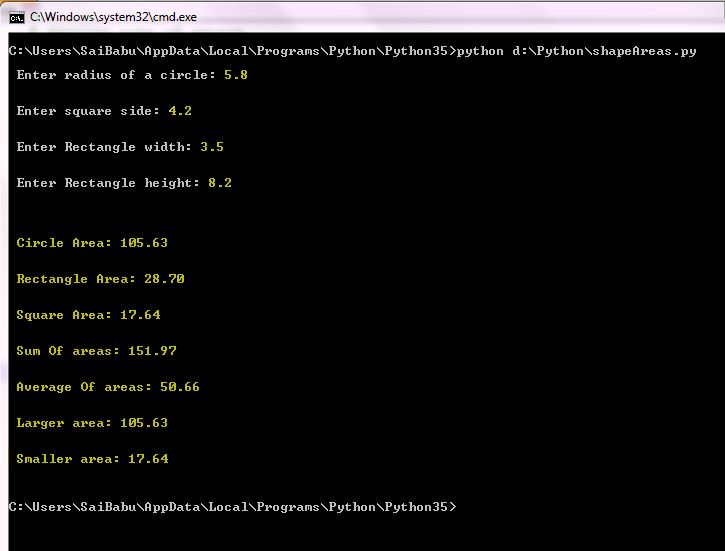# Answered! Write a program in Python that prompts the user for information (radius, side, height, width, etc) about at least three geometry shapes….

Write a program in Python that prompts the user for information (radius, side, height, width, etc) about at least three geometry shapes.

Possible geometry shapes: circle, ellipse, rectangle, square, equilateral triangle, right triangle, isosceles triangle, triangle, parallelogram, rhombus and trapezoid.

Don't use plagiarized sources. Get Your Custom Essay on
Answered! Write a program in Python that prompts the user for information (radius, side, height, width, etc) about at least three geometry shapes….
GET AN ESSAY WRITTEN FOR YOU FROM AS LOW AS \$13/PAGE

Use the information to:

– calculate the areas of these shapes;

– print the areas;

– print the sum of the areas;

– print the average of the areas;

– print the larger of the areas;

– print the smaller of the areas.

Hint: Python defines max and min functions that accept a sequence of values, each separated with comma.

Show the results on screen.

Remember: the user will only see what is on screen not what is inside the code. So, use clear and explanatory information.

For the coding, use:

– logic

– accuracy

– efficiency

Python Program:

class Circle:
“””
Circle Class
“””

def __init__(self, r=0):
“””
“””

def area(self):
“””
Function that calculates area of circle
“””

class Square:
“””
Square Class
“””

def __init__(self, s=0):
“””
Constructor that initializes side
“””
self.side = s;

def area(self):
“””
Function that calculates area of square
“””
return self.side * self.side;

class Rectangle:
“””
Rectangle Class
“””

def __init__(self, w=0, h=0):
“””
Constructor that initializes width and height
“””
self.width = w;
self.height = h;

def area(self):
“””
Function that calculates area of rectangle
“””
return self.width * self.height;

def main():
“””
Main Function
“””

# Creating Circle Class object

# Getting area of circle
circleArea = circleObj.area();

side = float(input(“nn Enter square side: “));

# Creating Square Class object
squareObj = Square(side);

# Getting area of square
squareArea = squareObj.area();

width = float(input(“nn Enter Rectangle width: “));
height = float(input(“nn Enter Rectangle height: “));

# Creating Rectangle Class object
rectangleObj = Rectangle(width, height);

# Getting area of rectangle
rectangleArea = rectangleObj.area();

# Storing all Areas in to a list
areas = [circleArea, squareArea, rectangleArea];

# Printing areas
print(“nnnn Circle Area: %.2f ” %(circleArea));
print(“nn Rectangle Area: %.2f ” %(rectangleArea));
print(“nn Square Area: %.2f ” %(squareArea));

# Printing results
print(“nn Sum Of areas: %.2f ” %(sum(areas)));
print(“nn Average Of areas: %.2f ” %(sum(areas)/float(len(areas))));
print(“nn Larger area: %.2f ” %(max(areas)));
print(“nn Smaller area: %.2f nn” %(min(areas)));

# Calling main function
main();

______________________________________________________________________________________________

Sample Output: